# Texas Go Math Grade 1 Lesson 1.4 Answer Key Tens and Ones to 50

Refer to our Texas Go Math Grade 1 Answer Key Pdf to score good marks in the exams. Test yourself by practicing the problems from Texas Go Math Grade 1 Lesson 1.4 Answer Key Tens and Ones to 50.

## Texas Go Math Grade 1 Lesson 1.4 Answer Key Tens and Ones to 50

Essential Question
How can you group cubes to show a number as tens and ones?
Explanation:
10 one cubes = 10
1 single cube = 1

Explore
Useto model the number.
Drawto show your work.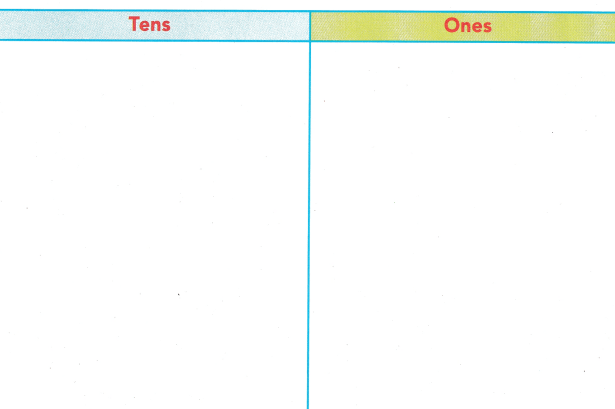For The Teacher

• Ask children to use 23 cubes and show them as tens and ones.
Explanation:
2 tens and 3 ones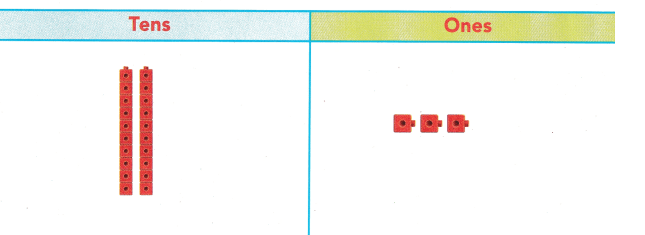Math Talk
Mathematical Processes
How did you figure out how many tens and ones are in 23? Explain.
Explanation:
2 tens blocks says as 20
3 ones blocks says as 3
20 + 3 = 23

Model and Draw

The 2 in 24 means 2 tens.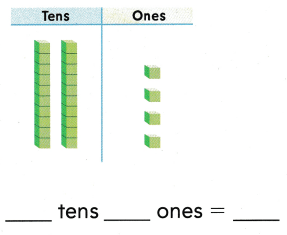Explanation:2 tens and 4 ones

The 2 in 42 means 2 ones.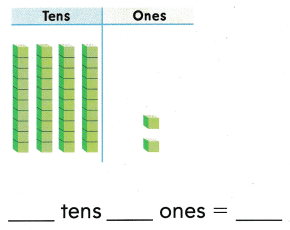Explanation:4 tens and 2 ones
42

Share and Show

Use your MathBoard andto show the tens and ones. Write the numbers.

Question 1.Explanation:
Counting the base ten blocks
tens and ones
3 tens and 5 ones = 35

Question 2.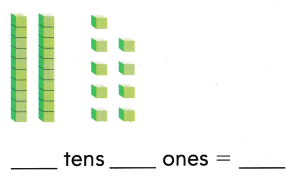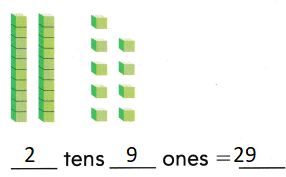Explanation:
Counting the base ten blocks
tens and ones
2 tens and 9 ones = 29

Question 3.Explanation:
Counting the base ten blocks
tens and ones
4 tens and 3 ones = 43

Question 4.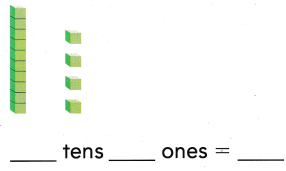Explanation:
Counting the base ten blocks
tens and ones
1 tens and 4 ones = 14

Problem Solving

Write the numbers.

Question 5.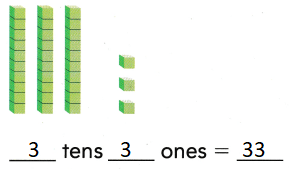Explanation:
Counting the base ten blocks
tens and ones
3 tens and 3 ones = 33

Question 6.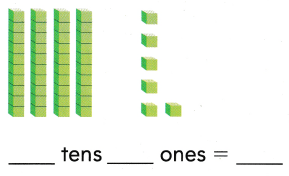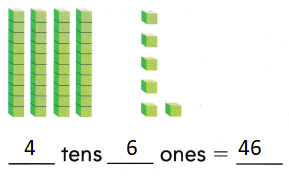Explanation:
Counting the base ten blocks
tens and ones
4 tens and 6 ones = 46

Question 7.Explanation:
Counting the base ten blocks
tens and ones
5 tens and 0 ones = 50

Question 8.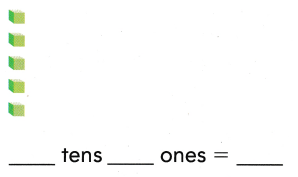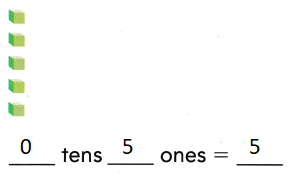Explanation:
Counting the base ten blocks
tens and ones
5 ones = 5

Solve. Write the numbers.

Question 9.
H.O.T. I am a number less than 50. I have 8 ones and some tens. What numbers could I be?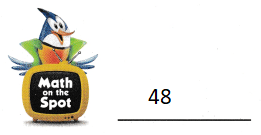Explanation:
I am a number less than 50. I have 8 ones and some tens.
4 tens and 8 ones = 48
48 < 50
48 is the number that could be.

Question 10.
Taylor wrote the number 13. Use mental math to tell what number has:Explanation:
1 ten in 13
10 + 3 = 13

Question 11.
Use Tools I have 24 cubes. Which shows how many tens and ones I have?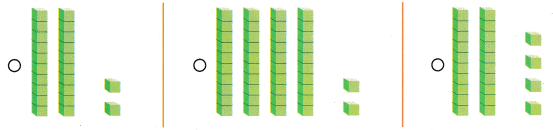Explanation:
2 tens and 4 ones
20 + 4 = 24

Question 12.
Hope has 2 sets of ten. She also has 5 ones. Which shows what Hope has?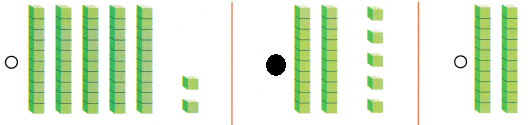Explanation:
2 tens and 5 ones
20 + 5 = 25

Question 13.
Texas Test Prep Use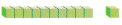to show the tens and ones. Which number does the model show?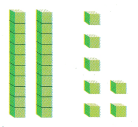(A) 9
(B) 72
(C) 27
Explanation:
2 tens and 7 ones = 27

Take Home Activity

• Write a two-digit number from 20 to 50, such as 26. Ask your child to tell which digit names the tens and which digit names the ones. Repeat with different numbers.
Explanation:
a two-digit number from 20 to 50, = 35
3 is tens and 5 is ones

### Texas Go Math Grade 1 Lesson 1.4 Homework and Practice Answer Key

Write the numbers.

Question 1.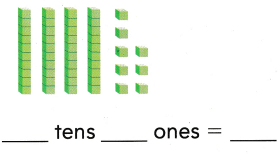Explanation:
Counting the base ten blocks
tens and ones
4 tens and 8 ones = 48

Question 2.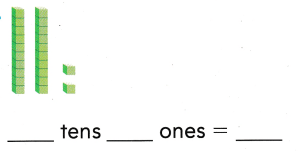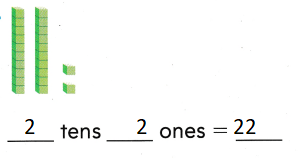Explanation:
Counting the base ten blocks
tens and ones
2 tens and 2 ones = 22

Question 3.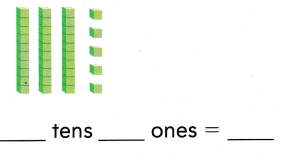Explanation:
Counting the base ten blocks
tens and ones
3 tens and 5 ones = 35

Question 4.Explanation:
Counting the base ten blocks
tens and ones
6 tens and 0 ones = 60

Problem Solving

Question 5.
Kim wrote the number 11. Use mental math to tell what number has: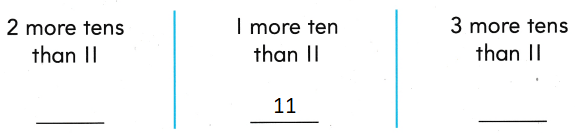Explanation:
1 ten in 11
10 + 1 = 11

Texas Test Prep

Lesson Check

Question 6.
There are 26 letters in the alphabet? Which shows the number 26?Explanation:
2 tens and 6 ones = 26
20 + 6 = 26

Question 7.
Al put 72 paper clips together to make a long chain. How many paper clips did he use in all?
(A) 7 tens 2 ones
(B) 2 tens 7 ones
(C) 1 ten 72 ones
Explanation:
7 tens 2 ones
72 paper clips that he use in all

Question 8.
Bart has 3 sets of ten. He also has I one. Which model shows Barfs number?Explanation:
3 tens and 1 ones = 31
30 + 1 = 31

Question 9.
Multi-Step Jin has between 1 and 3 tens. Carla has between 4 and 6 ones. What number can they make?
(A) 13
(B) 25
(C) 46